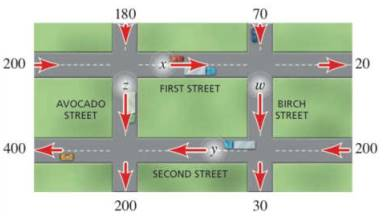Chapter 11.1, Problem 74E### Algebra and Trigonometry (MindTap ...

4th Edition
James Stewart + 2 others
ISBN: 9781305071742

#### Solutions

Chapter
Section### Algebra and Trigonometry (MindTap ...

4th Edition
James Stewart + 2 others
ISBN: 9781305071742
Textbook Problem

# APPLICATIONSTraffic Flow A section of a city’s street network is shown in the figure. The arrows indicate one-way streets, and the numbers show how many cars enter or leave this section of the city via the indicated street in a certain one-hour period. The variables x, y, z, and w represent the number of cars that travel along the portions of First, Second, Avocado, and Birch Streets during this period. Find x, y, z, and w, assuming that none of the cars stop or park on any of the streets shown.To determine

To find:

The values of x, y, z, and w.

Explanation

Given:

A section of a city’s street network is shown in the figure. The arrows indicate one-way streets, and the numbers show how many cars enter or leave this section of the city via the indicated street in a certain one-hour period. The variables x, y, z, and w represent the number of cars that travel along the portions of First, Second, Avocado, and Birch Streets during this period.

Figure (1)

Calculation:

The variables x, y, z, and w represents the number of cars that travel along the portions of First, Second, Avocado, and Birch Streets during this period.

The system of equations is given below.

200+180xz=070+x20w=0200+w30y=0z+y400200=0

Consider the system of equations,

{200+180xz=070+x20w=0200+w30y=0z+y400200=0

Or,

{x+z=380xw=50wy=170z+y=600

The augmented matrix obtained is,

.

Transform the augmented matrix into row echelon form.

Perform the transformation R2R2R1.



Perform the transformation R2R4.



Perform the transformation R3R3+R2

### Still sussing out bartleby?

Check out a sample textbook solution.

See a sample solution

#### The Solution to Your Study Problems

Bartleby provides explanations to thousands of textbook problems written by our experts, many with advanced degrees!

Get Started